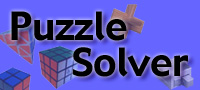Puzzle of the MonthPuzzle StoreSimilar Puzzles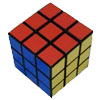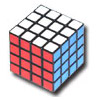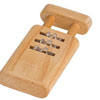# The Brain Puzzle Solution

The object of this puzzle is to get all the sliders either in or out. Several stacked and slotted disks inside the puzzle limit the range of movement at each step in the puzzle.IN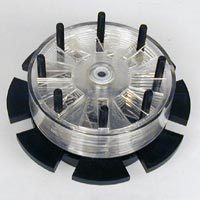OUT

This puzzle is easy because at any point in the solution there are only two sliders that can move (the previous one in the sequence and the next one in the sequence). So as long as you never move the same slider twice in a row, you will eventually solve the puzzle. The real objective of this puzzle is to solve it fast. To do this you need to memorize the solution.

The sequence of moves between the two states is pretty easy to learn. Each slider has a number printed on top (1 through 8). In the solution, every 2nd move is slider 1. Every 4th move is slider 2. Evey 8th move is slider 3. Every 16th move is slider 4 and so on.

Here is the total sequence from all sliders in to all siders out. To go the other direction do this sequence of moves backwards:

Step 1:    1
Step 2:    2 1
Step 3:    3 121
Step 4:    4 1213121
Step 5:    5 121312141213121
Step 6:    6 1213121412131215121312141213121
Step 7:    7 121312141213121512131214121312161213121412131215121312141213121
Step 8:    8 121312141213121512131214121312161213121412Site Info